Next: Lagrangian mechanics Up: Rotating reference frames Previous: Roche radius

# Exercises

1. A ball bearing is dropped down an elevator shaft in the Empire State Building (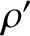m, latitude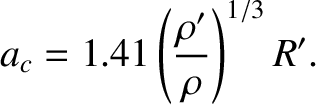N). Find the ball bearing's horizontal deflection (magnitude and direction) at the bottom of the shaft due to the Coriolis force . Neglect air resistance. (Modified from Fowles and Cassiday 2005.)

2. A projectile is fired due east, at an elevation angle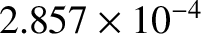, from a point on the Earth's surface whose latitude is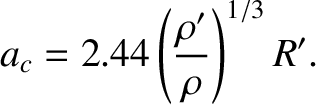. Demonstrate that the projectile strikes the ground with a lateral deflection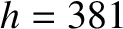. Is the deflection northward or southward? Here,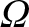is the Earth's angular velocity,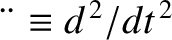the projectile's initial speed, and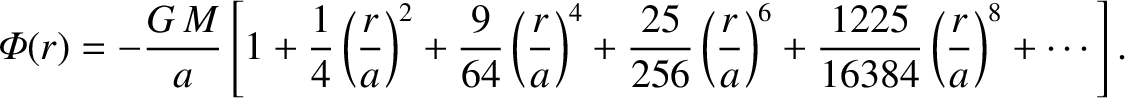the acceleration due to gravity. Neglect air resistance. (Modified from Thornton and Marion 2004.)

3. A particle is thrown vertically upward, reaches some maximum height, and falls back to the ground. Show that the horizontal Coriolis deflection of the particle when it returns to the ground is opposite in direction, and four times greater in magnitude, than the Coriolis deflection when it is dropped at rest from the same maximum height. Neglect air resistance. (From Goldstein, et al. 2002.)

4. A ball of massrolls without friction over a horizontal plane located on the surface of the Earth. Show that in the northern hemisphere it rolls in a clockwise sense (seen from above) around a circle of radius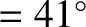where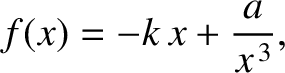is the speed of the ball,the Earth's angular velocity, and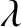the terrestrial latitude.

5. A satellite is in a circular orbit of radiusabout the Earth. Let us define a set of co-moving Cartesian coordinates, centered on the satellite, such that the-axis always points toward the center of the Earth, the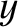-axis in the direction of the satellite's orbital motion, and the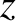-axis in the direction of the satellite's orbital angular velocity,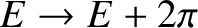. Demonstrate that the equation of motion of a small mass in orbit about the satellite are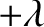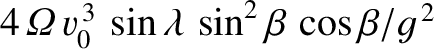and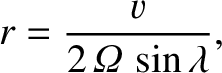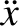assuming that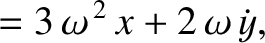and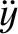. Neglect the gravitational attraction between the satellite and the mass. Show that the mass executes a retrograde (i.e., in the opposite sense to the satellite's orbital rotation) elliptical orbit about the satellite whose period matches that of the satellite's orbit, and whose major and minor axes are in the ratio, and are aligned along the- and-axes, respectively.

6. Treating the Earth as a uniform-density, liquid spheroid, and taking rotational flattening into account, show that the variation of the surface acceleration,, with terrestrial latitude,, is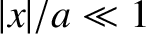Here,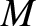is the terrestrial mass,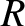the mean terrestrial radius, andthe terrestrial axial angular velocity.

7. The Moon's mean orbital period about the Earth is approximately 27.32 days, and its orbital motion is in the same direction as the Earth's axial rotation (whose period is 24 hours). Use this data to show that, on average, high tides at a given point on the Earth occur every 12 hours and 27 minutes.

8. Demonstrate that the mean time interval between successive spring tides is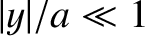days.

9. Let us model the Earth as a completely rigid sphere that is covered by a shallow ocean of negligible density. Demonstrate that the tidal elongation of the ocean layer due to the Moon is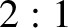whereis the mass of the Earth,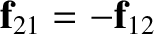the mass of the Moon,the radius of the Earth, andthe radius of the lunar orbit. Show that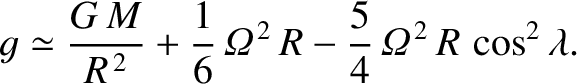, and also that the tidal elongation of the ocean layer due to the Sun is such that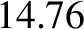.

10. Estimate the tidal heating rate of the Earth due to the Moon. Is this rate significant compared to the net heating rate from solar radiation?

11. Estimate the tidal elongation of the Sun due to the Earth.

12. An approximately spherical moon of uniform density, mass, radius, and effective rigidity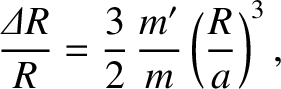, is in a circular orbit of major radiusabout a spherical planet of mass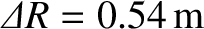. The moon rotates about an axis passing through its center of mass that is directed normal to the orbital plane. Suppose that the moon is in a synchronous state such that its rotational angular velocity,, matches its orbital angular velocity,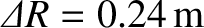. Let,,be a set of Cartesian coordinates, centered on the moon, such that the-axis is normal to the orbital plane, and the-axis is directed from the center of the moon to the center of the planet. Assuming that the moon responds elastically to the centrifugal and tidal potentials, show that the changes in radius, parallel to the three coordinate axes, induced by the centrifugal potential are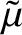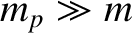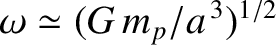and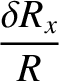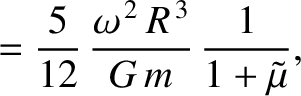whereas the corresponding changes induced by the tidal potential are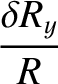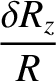and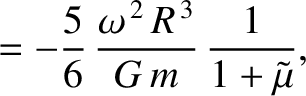Assuming that these changes are additive, deduce that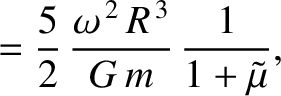Estimate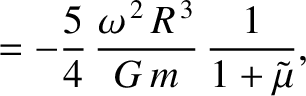,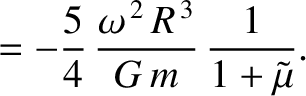, and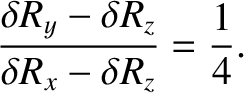for the Earth's Moon (which is in a synchronous state).

13. An artificial satellite consists of two point objects of mass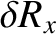connected by a light rigid rod of length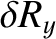. The satellite is placed in a circular orbit of radius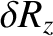(measured from the mid-point of the rod) around a planet of mass. The rod is oriented such that it always points toward the center of the planet. Demonstrate that the tension in the rod is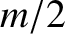Next: Lagrangian mechanics Up: Rotating reference frames Previous: Roche radius
Richard Fitzpatrick 2016-03-31